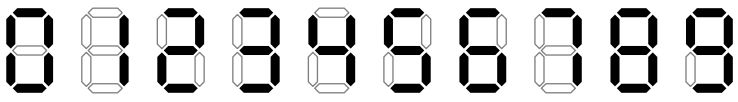시간 제한메모리 제한제출정답맞힌 사람정답 비율
2 초 512 MB12000.000%

## 문제

Rumor has it that IOI 2048 is going to be held in Innopolis! In this regard, a large display counter was installed in Innopolis, indicating the number of nanoseconds before the start of the Olympiad! Initially, a certain number was set to be displayed, and every nanosecond this number decreases by one. Leading zeros are not displayed.

The digits are displayed using standard seven-segment indicators. The way the digits look is shown in the picture:Nanoseconds change so quickly that it’s almost impossible to see the number the display shows at the moment. But, by connecting a high-precision sensor attached to the power cord of the display, it was possible to obtain the values $a_i$ — the number of segments turned on during the each of $n$ nanoseconds in a row. Since there is still time before IOI 2048, the number on the timer at any time was positive.

Write a program that calculates the number of possible initial values (corresponding to the measurement $a_1$), and any $m$ of these values. If the number of possible initial values are less than $m$, you should print all of them.

## 입력

The first line contains two numbers $n$ and $m$ ($1 \le n \le 10^5$, $0 \le m \le 10$) — the number of nanoseconds and the number of values to print. The next line contains $n$ integers $a_i$ ($2 \le a_i \le 1000$) — the number of segments that are turned on during the $i$-th nanosecond.

## 출력

In the first line print $k$ — the number of possible initial values of the counter modulo $1\,000\,000\,007$. Then, print $m$ different initial values of the number on the display, consistent with the given measurements. If the actual number of values (before taking it modulo $1\,000\,000\,007$) is less than $m$, print all the suitable values. You can print these numbers in any order, each one in a separate line.

## 서브태스크

번호배점제한
117

$n = 1$, $m = 0$, $a_i \le 100$

210

$n = 1$, $m \le 10$, $a_i \le 100$

319

$n \le 100$, $a_i \le 10$

422

$n \le 100$, $a_i \le 20$

532

## 예제 입력 1

5 1
11 15 14 15 11


## 예제 출력 1

3
1151


## 예제 입력 2

10 1
13 10 14 12 13 9 12 11 10 11


## 예제 출력 2

1
102


## 예제 입력 3

4 10
29 28 29 29


## 예제 출력 3

108637
448765
812225
19541715
417773115
161177415
117347175
206215
8127315
12761415
54110175


## 예제 입력 4

1 6
6


## 예제 출력 4

6
111
41
14
6
77
9


## 힌트

In the first example, if initial value on the display is 1151, then it changes in the following way:

Number on the display Number of segments
1151 $2 + 2 + 5 + 2 = 11$
1150 $2 + 2 + 5 + 6 = 15$
1149 $2 + 2 + 4 + 6 = 14$
1148 $2 + 2 + 4 + 7 = 15$
1147 $2 + 2 + 4 + 3 = 11$

Other possible initial values are 451 and 761, you can print any of them.

## 채점 및 기타 정보

• 예제는 채점하지 않는다.